Thermodynamics

# Statistical Interpretation of Entropy and the Second Law of Thermodynamics: The Underlying Explanation

OpenStaxCollege

### Learning Objectives

• Identify probabilities in entropy.
• Analyze statistical probabilities in entropic systems.
When you toss a coin a large number of times, heads and tails tend to come up in roughly equal numbers. Why doesn’t heads come up 100, 90, or even 80% of the time? (credit: Jon Sullivan, PDPhoto.org)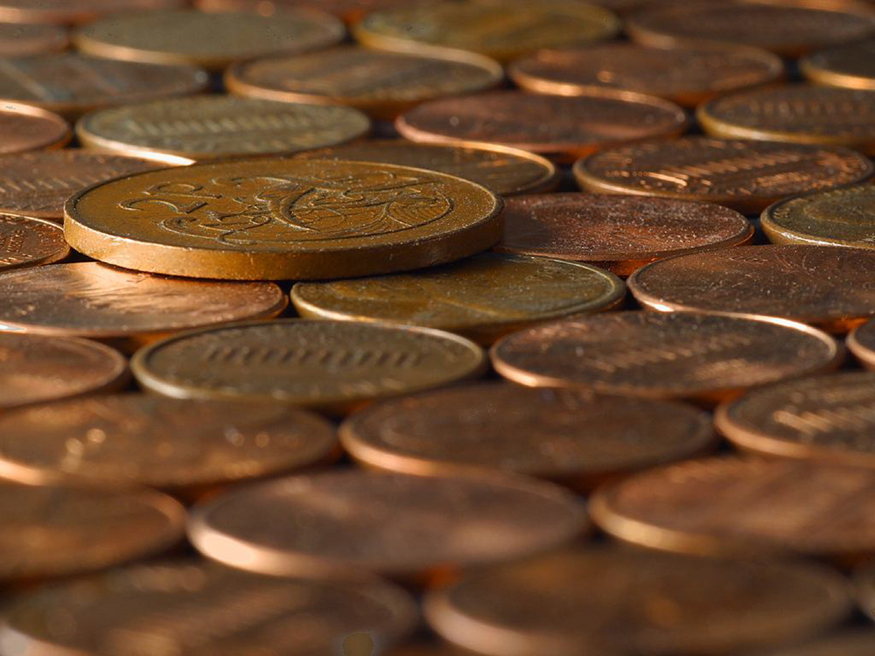The various ways of formulating the second law of thermodynamics tell what happens rather than why it happens. Why should heat transfer occur only from hot to cold? Why should energy become ever less available to do work? Why should the universe become increasingly disorderly? The answer is that it is a matter of overwhelming probability. Disorder is simply vastly more likely than order.

When you watch an emerging rain storm begin to wet the ground, you will notice that the drops fall in a disorganized manner both in time and in space. Some fall close together, some far apart, but they never fall in straight, orderly rows. It is not impossible for rain to fall in an orderly pattern, just highly unlikely, because there are many more disorderly ways than orderly ones. To illustrate this fact, we will examine some random processes, starting with coin tosses.

# Coin Tosses

What are the possible outcomes of tossing 5 coins? Each coin can land either heads or tails. On the large scale, we are concerned only with the total heads and tails and not with the order in which heads and tails appear. The following possibilities exist: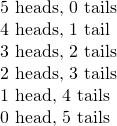These are what we call macrostates. A macrostate is an overall property of a system. It does not specify the details of the system, such as the order in which heads and tails occur or which coins are heads or tails.

Using this nomenclature, a system of 5 coins has the 6 possible macrostates just listed. Some macrostates are more likely to occur than others. For instance, there is only one way to get 5 heads, but there are several ways to get 3 heads and 2 tails, making the latter macrostate more probable. [link] lists of all the ways in which 5 coins can be tossed, taking into account the order in which heads and tails occur. Each sequence is called a microstate—a detailed description of every element of a system.

5-Coin Toss
Individual microstates Number of microstates
5 heads, 0 tails HHHHH 1
4 heads, 1 tail HHHHT, HHHTH, HHTHH, HTHHH, THHHH 5
3 heads, 2 tails HTHTH, THTHH, HTHHT, THHTH, THHHT HTHTH, THTHH, HTHHT, THHTH, THHHT 10
2 heads, 3 tails TTTHH, TTHHT, THHTT, HHTTT, TTHTH, THTHT, HTHTT, THTTH, HTTHT, HTTTH 10
1 head, 4 tails TTTTH, TTTHT, TTHTT, THTTT, HTTTT 5
0 heads, 5 tails TTTTT 1
Total: 32

The macrostate of 3 heads and 2 tails can be achieved in 10 ways and is thus 10 times more probable than the one having 5 heads. Not surprisingly, it is equally probable to have the reverse, 2 heads and 3 tails. Similarly, it is equally probable to get 5 tails as it is to get 5 heads. Note that all of these conclusions are based on the crucial assumption that each microstate is equally probable. With coin tosses, this requires that the coins not be asymmetric in a way that favors one side over the other, as with loaded dice. With any system, the assumption that all microstates are equally probable must be valid, or the analysis will be erroneous.

The two most orderly possibilities are 5 heads or 5 tails. (They are more structured than the others.) They are also the least likely, only 2 out of 32 possibilities. The most disorderly possibilities are 3 heads and 2 tails and its reverse. (They are the least structured.) The most disorderly possibilities are also the most likely, with 20 out of 32 possibilities for the 3 heads and 2 tails and its reverse. If we start with an orderly array like 5 heads and toss the coins, it is very likely that we will get a less orderly array as a result, since 30 out of the 32 possibilities are less orderly. So even if you start with an orderly state, there is a strong tendency to go from order to disorder, from low entropy to high entropy. The reverse can happen, but it is unlikely.

100-Coin Toss
Macrostate Number of microstates
Heads Tails (W)
100 0 1
99 1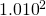95 5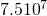90 10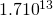75 25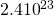60 40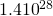55 45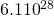51 49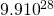50 50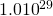49 5145 5540 6025 7510 905 951 990 100 1
Total: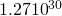This result becomes dramatic for larger systems. Consider what happens if you have 100 coins instead of just 5. The most orderly arrangements (most structured) are 100 heads or 100 tails. The least orderly (least structured) is that of 50 heads and 50 tails. There is only 1 way (1 microstate) to get the most orderly arrangement of 100 heads. There are 100 ways (100 microstates) to get the next most orderly arrangement of 99 heads and 1 tail (also 100 to get its reverse). And there are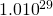ways to get 50 heads and 50 tails, the least orderly arrangement. [link] is an abbreviated list of the various macrostates and the number of microstates for each macrostate. The total number of microstates—the total number of different ways 100 coins can be tossed—is an impressively large. Now, if we start with an orderly macrostate like 100 heads and toss the coins, there is a virtual certainty that we will get a less orderly macrostate. If we keep tossing the coins, it is possible, but exceedingly unlikely, that we will ever get back to the most orderly macrostate. If you tossed the coins once each second, you could expect to get either 100 heads or 100 tails once in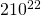years! This period is 1 trillion (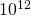) times longer than the age of the universe, and so the chances are essentially zero. In contrast, there is an 8% chance of getting 50 heads, a 73% chance of getting from 45 to 55 heads, and a 96% chance of getting from 40 to 60 heads. Disorder is highly likely.

# Disorder in a Gas

The fantastic growth in the odds favoring disorder that we see in going from 5 to 100 coins continues as the number of entities in the system increases. Let us now imagine applying this approach to perhaps a small sample of gas. Because counting microstates and macrostates involves statistics, this is called statistical analysis. The macrostates of a gas correspond to its macroscopic properties, such as volume, temperature, and pressure; and its microstates correspond to the detailed description of the positions and velocities of its atoms. Even a small amount of gas has a huge number of atoms: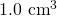of an ideal gas at 1.0 atm and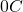has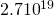atoms. So each macrostate has an immense number of microstates. In plain language, this means that there are an immense number of ways in which the atoms in a gas can be arranged, while still having the same pressure, temperature, and so on.

The most likely conditions (or macrostates) for a gas are those we see all the time—a random distribution of atoms in space with a Maxwell-Boltzmann distribution of speeds in random directions, as predicted by kinetic theory. This is the most disorderly and least structured condition we can imagine. In contrast, one type of very orderly and structured macrostate has all of the atoms in one corner of a container with identical velocities. There are very few ways to accomplish this (very few microstates corresponding to it), and so it is exceedingly unlikely ever to occur. (See [link](b).) Indeed, it is so unlikely that we have a law saying that it is impossible, which has never been observed to be violated—the second law of thermodynamics.

(a) The ordinary state of gas in a container is a disorderly, random distribution of atoms or molecules with a Maxwell-Boltzmann distribution of speeds. It is so unlikely that these atoms or molecules would ever end up in one corner of the container that it might as well be impossible. (b) With energy transfer, the gas can be forced into one corner and its entropy greatly reduced. But left alone, it will spontaneously increase its entropy and return to the normal conditions, because they are immensely more likely.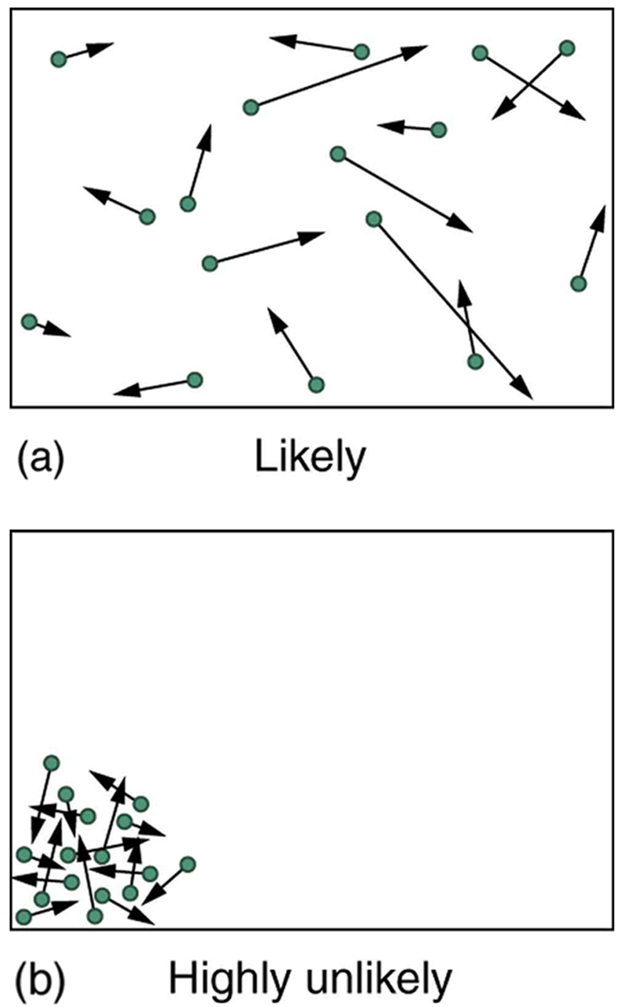The disordered condition is one of high entropy, and the ordered one has low entropy. With a transfer of energy from another system, we could force all of the atoms into one corner and have a local decrease in entropy, but at the cost of an overall increase in entropy of the universe. If the atoms start out in one corner, they will quickly disperse and become uniformly distributed and will never return to the orderly original state ([link](b)). Entropy will increase. With such a large sample of atoms, it is possible—but unimaginably unlikely—for entropy to decrease. Disorder is vastly more likely than order.

The arguments that disorder and high entropy are the most probable states are quite convincing. The great Austrian physicist Ludwig Boltzmann (1844–1906)—who, along with Maxwell, made so many contributions to kinetic theory—proved that the entropy of a system in a given state (a macrostate) can be written as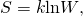where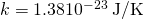is Boltzmann’s constant, and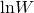is the natural logarithm of the number of microstates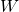corresponding to the given macrostate.is proportional to the probability that the macrostate will occur. Thus entropy is directly related to the probability of a state—the more likely the state, the greater its entropy. Boltzmann proved that this expression for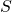is equivalent to the definition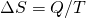, which we have used extensively.

Thus the second law of thermodynamics is explained on a very basic level: entropy either remains the same or increases in every process. This phenomenon is due to the extraordinarily small probability of a decrease, based on the extraordinarily larger number of microstates in systems with greater entropy. Entropy can decrease, but for any macroscopic system, this outcome is so unlikely that it will never be observed.

Entropy Increases in a Coin Toss

Suppose you toss 100 coins starting with 60 heads and 40 tails, and you get the most likely result, 50 heads and 50 tails. What is the change in entropy?

Strategy

Noting that the number of microstates is labeledin [link] for the 100-coin toss, we can use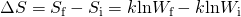to calculate the change in entropy.

Solution

The change in entropy is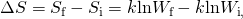where the subscript i stands for the initial 60 heads and 40 tails state, and the subscript f for the final 50 heads and 50 tails state. Substituting the values forfrom [link] gives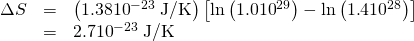Discussion

This increase in entropy means we have moved to a less orderly situation. It is not impossible for further tosses to produce the initial state of 60 heads and 40 tails, but it is less likely. There is about a 1 in 90 chance for that decrease in entropy (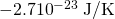) to occur. If we calculate the decrease in entropy to move to the most orderly state, we get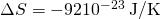. There is about a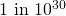chance of this change occurring. So while very small decreases in entropy are unlikely, slightly greater decreases are impossibly unlikely. These probabilities imply, again, that for a macroscopic system, a decrease in entropy is impossible. For example, for heat transfer to occur spontaneously from 1.00 kg of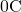ice to itsenvironment, there would be a decrease in entropy of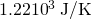. Given that a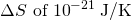corresponds to about achance, a decrease of this size (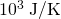) is an utter impossibility. Even for a milligram of melted ice to spontaneously refreeze is impossible.

Problem-Solving Strategies for Entropy
1. Examine the situation to determine if entropy is involved.
2. Identify the system of interest and draw a labeled diagram of the system showing energy flow.
3. Identify exactly what needs to be determined in the problem (identify the unknowns). A written list is useful.
4. Make a list of what is given or can be inferred from the problem as stated (identify the knowns). You must carefully identify the heat transfer, if any, and the temperature at which the process takes place. It is also important to identify the initial and final states.
5. Solve the appropriate equation for the quantity to be determined (the unknown). Note that the change in entropy can be determined between any states by calculating it for a reversible process.
6. Substitute the known value along with their units into the appropriate equation, and obtain numerical solutions complete with units.
7. To see if it is reasonable: Does it make sense? For example, total entropy should increase for any real process or be constant for a reversible process. Disordered states should be more probable and have greater entropy than ordered states.

# Section Summary

• Disorder is far more likely than order, which can be seen statistically.
• The entropy of a system in a given state (a macrostate) can be written as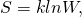where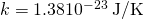is Boltzmann’s constant, and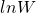is the natural logarithm of the number of microstatescorresponding to the given macrostate.

# Conceptual Questions

Explain why a building made of bricks has smaller entropy than the same bricks in a disorganized pile. Do this by considering the number of ways that each could be formed (the number of microstates in each macrostate).

# Problem Exercises

Using [link], verify the contention that if you toss 100 coins each second, you can expect to get 100 heads or 100 tails once inyears; calculate the time to two-digit accuracy.

It should happen twice in every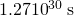or once in every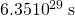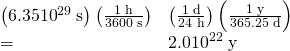What percent of the time will you get something in the range from 60 heads and 40 tails through 40 heads and 60 tails when tossing 100 coins? The total number of microstates in that range is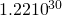. (Consult [link].)

(a) If tossing 100 coins, how many ways (microstates) are there to get the three most likely macrostates of 49 heads and 51 tails, 50 heads and 50 tails, and 51 heads and 49 tails? (b) What percent of the total possibilities is this? (Consult [link].)

(a)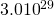(b) 24%

(a) What is the change in entropy if you start with 100 coins in the 45 heads and 55 tails macrostate, toss them, and get 51 heads and 49 tails? (b) What if you get 75 heads and 25 tails? (c) How much more likely is 51 heads and 49 tails than 75 heads and 25 tails? (d) Does either outcome violate the second law of thermodynamics?

(a) What is the change in entropy if you start with 10 coins in the 5 heads and 5 tails macrostate, toss them, and get 2 heads and 8 tails? (b) How much more likely is 5 heads and 5 tails than 2 heads and 8 tails? (Take the ratio of the number of microstates to find out.) (c) If you were betting on 2 heads and 8 tails would you accept odds of 252 to 45? Explain why or why not.

(a)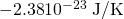(b) 5.6 times more likely

(c) If you were betting on two heads and 8 tails, the odds of breaking even are 252 to 45, so on average you would break even. So, no, you wouldn’t bet on odds of 252 to 45.

10-Coin Toss
Macrostate Number of Microstates (W)
Heads Tails
10 0 1
9 1 10
8 2 45
7 3 120
6 4 210
5 5 252
4 6 210
3 7 120
2 8 45
1 9 10
0 10 1
Total: 1024

(a) If you toss 10 coins, what percent of the time will you get the three most likely macrostates (6 heads and 4 tails, 5 heads and 5 tails, 4 heads and 6 tails)? (b) You can realistically toss 10 coins and count the number of heads and tails about twice a minute. At that rate, how long will it take on average to get either 10 heads and 0 tails or 0 heads and 10 tails?

(a) Construct a table showing the macrostates and all of the individual microstates for tossing 6 coins. (Use [link] as a guide.) (b) How many macrostates are there? (c) What is the total number of microstates? (d) What percent chance is there of tossing 5 heads and 1 tail? (e) How much more likely are you to toss 3 heads and 3 tails than 5 heads and 1 tail? (Take the ratio of the number of microstates to find out.)

(b) 7

(c) 64

(d) 9.38%

(e) 3.33 times more likely (20 to 6)

In an air conditioner, 12.65 MJ of heat transfer occurs from a cold environment in 1.00 h. (a) What mass of ice melting would involve the same heat transfer? (b) How many hours of operation would be equivalent to melting 900 kg of ice? (c) If ice costs 20 cents per kg, do you think the air conditioner could be operated more cheaply than by simply using ice? Describe in detail how you evaluate the relative costs.

## Glossary

macrostate
an overall property of a system
microstate
each sequence within a larger macrostate
statistical analysis
using statistics to examine data, such as counting microstates and macrostates

## LicenseStatistical Interpretation of Entropy and the Second Law of Thermodynamics: The Underlying Explanation by OpenStaxCollege is licensed under a Creative Commons Attribution 4.0 International License, except where otherwise noted.